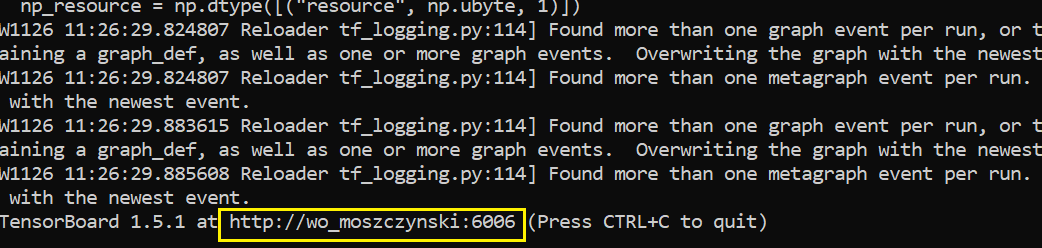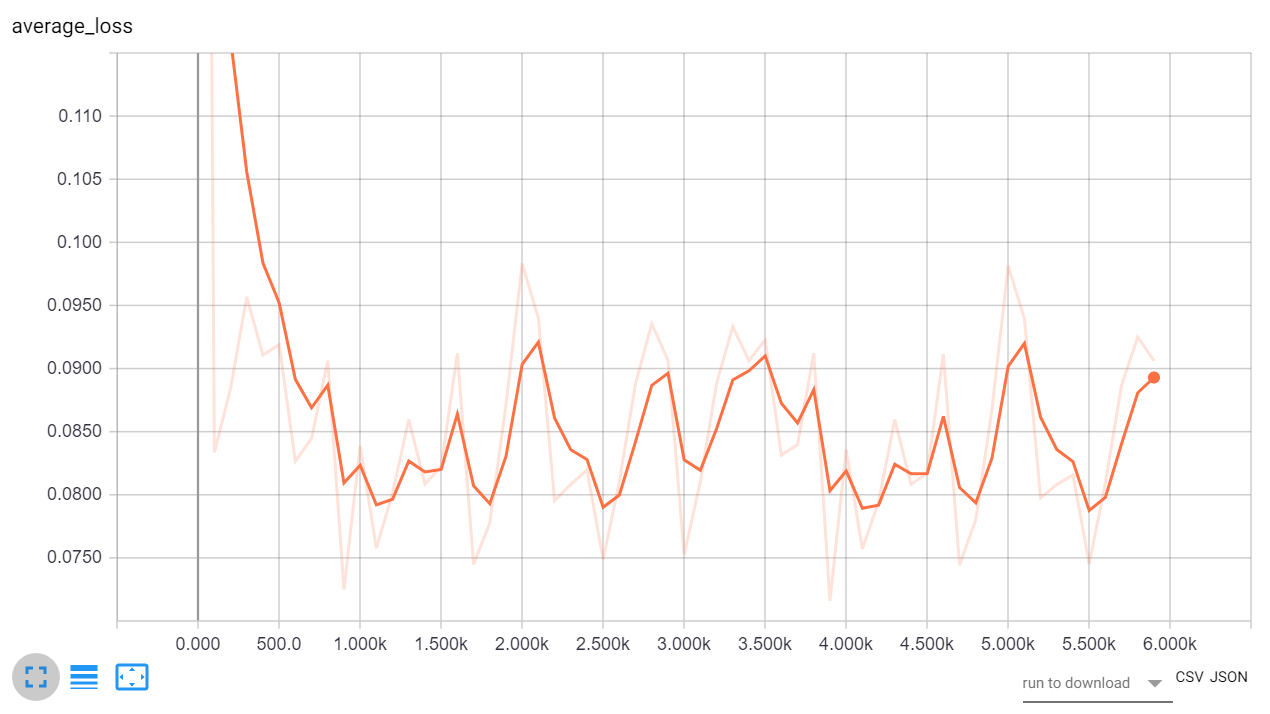# Linear regression with TensorFlow

## Part one: Numpy method

In :
```import pandas as pd
import tensorflow as tf
import itertools
```
` `

## Combined Cycle Power Plant Data Set

#### Data Set Information:

The dataset contains 9568 data points collected from a Combined Cycle Power Plant over 6 years (2006-2011), when the power plant was set to work with full load. Features consist of hourly average ambient variables Temperature (T), Ambient Pressure (AP), Relative Humidity (RH) and Exhaust Vacuum (V) to predict the net hourly electrical energy output (EP) of the plant.
A combined cycle power plant (CCPP) is composed of gas turbines (GT), steam turbines (ST) and heat recovery steam generators. In a CCPP, the electricity is generated by gas and steam turbines, which are combined in one cycle, and is transferred from one turbine to another. While the Vacuum is colected from and has effect on the Steam Turbine, he other three of the ambient variables effect the GT performance.
For comparability with our baseline studies, and to allow 5×2 fold statistical tests be carried out, we provide the data shuffled five times. For each shuffling 2-fold CV is carried out and the resulting 10 measurements are used for statistical testing.
We provide the data both in .ods and in .xlsx formats.

#### Attribute Information:

Features consist of hourly average ambient variables

• Temperature (T) in the range 1.81°C and 37.11°C,
• Ambient Pressure (AP) in the range 992.89-1033.30 milibar,
• Relative Humidity (RH) in the range 25.56% to 100.16%
• Exhaust Vacuum (V) in teh range 25.36-81.56 cm Hg
• Net hourly electrical energy output (EP) 420.26-495.76 MW
The averages are taken from various sensors located around the plant that record the ambient variables every second. The variables are given without normalization.

### Step 1: prepare the data

In [ ]:
```df = pd.read_csv('c:/1/Folds5x2_pp.csv')
df.sample(3)
```
In [ ]:
```del df['Unnamed: 0']
df.columns
```
In [ ]:
```df.columns = ['Temperature', 'Exhaust_Vacuum', 'Ambient_Pressure', 'Relative_Humidity', 'Energy_output']
df.sample(3)
```

### Step 2: Convert Data

We convert numeric variables in the correct Tensorflow format. Tensorflow provides a continuous variable conversion method: tf.feature_column.numeric_column ().

Separation of a column into an independent variable and a dependent variable.

In [ ]:
```FEATURES = ['Temperature', 'Exhaust_Vacuum', 'Ambient_Pressure', 'Relative_Humidity']
LABEL = 'Energy_output'
```
In [ ]:
```Ewa = [tf.feature_column.numeric_column(k) for k in FEATURES]
Ewa
```

### Step 3: Defining the estimator

Tensorflow will automatically create a file called “train2” in your working directory. You must use this path to access Tensorboard. The estimator applies to independent variables.

In [ ]:
```estimator = tf.estimator.LinearRegressor(
feature_columns=Ewa,
model_dir="train2")
```

To instruct Tensorflow how to feed the model, you can use pandas_input_fn. This object needs 5 parameters:
x: function data y: label data batch_size: batch. Default 128 num_epoch: by default number of epochs 1 random: Random or not data. Default None

In [ ]:
```def get_input_fn(data_set, num_epochs=None, n_batch = 128, shuffle=True):
return tf.estimator.inputs.pandas_input_fn(
x=pd.DataFrame({k: data_set[k].values for k in FEATURES}),
y = pd.Series(data_set[LABEL].values),
batch_size=n_batch,
num_epochs=num_epochs,
shuffle=shuffle)
```

### Step 4: Model training

``````- To feed the model you can use the function created above: get_input_fn.
- Then you instruct the model to iterate 1000 times.
- Remember that you do not specify the number of epochs (num_epochs).
- It is better to set the number of epochs to none and define the number of iterations.

``````

To test the model, we must divide the data set into a test set and a training set.

In [ ]:
```df_train=df.sample(frac=0.8,random_state=200)
df_test=df.drop(df_train.index)
print(df_train.shape, df_test.shape)
```
In [ ]:
```estimator.train(input_fn=get_input_fn(df_train,
num_epochs=None,
n_batch = 356,
shuffle=False),
steps=1000)
```

We check the CMD TensorBoard command console.

##### tensorboard –logdir=.trainlinreg

Tensorboard is located in this URL: http://localhost:6006

It could also be located at the following location.

http://wo_moszczynski:6006## Step 5. Model assessment

To enter a test set, use the following code:

In [ ]:
```ev = estimator.evaluate(
input_fn=get_input_fn(df_test,
num_epochs=1,
n_batch = 356,
shuffle=False))
```

Print the loss using by the code below:

In [ ]:
```average_loss = ev["average_loss"]
print("average_loss: ",format(average_loss))
```
In [ ]:
```loss_score = ev["loss"]
print("Loss: {0:f}".format(loss_score))
```

The model has a average loss of 26. You can check the summary statistics to find out how big the error is.

In [ ]:
```df_test['Energy_output'].describe()
```
In [ ]:
```PKP=(average_loss/ df_test['Energy_output'].mean())*100
print('Average error in relation to the average value: ',PKP)
```

## Step 6. Making a forecast

Making a forecast is based on the fact that we have a model and we have a set of independent variables. Now we substitute the independent variables into the model and get the result. We will create 4 random variables and make a forecast for these records.

##### We create a sample of 4 records without output variables.
In [ ]:
```import numpy as np

sample4 =df.sample(4)
result = sample4['Energy_output'].copy() ## <= to have a comparison later
sample4['Energy_output']=np.nan
sample4
```
In [ ]:
```y = estimator.predict(
input_fn=get_input_fn(sample4,
num_epochs=1,
n_batch = 256,
shuffle=False))
```
In [ ]:
```predictions = list(p["predictions"] for p in itertools.islice(y, 4))
print("Predictions: {}".format(str(predictions)))
```
In [ ]:
```predictions
```

I’m converting array to dataframe

In [ ]:
```conc = np.vstack(predictions)
conc
```
In [ ]:
```newdf = pd.DataFrame(conc)
newdf
```
In [ ]:
```result
```